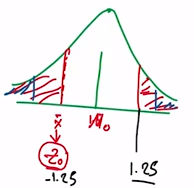Need Help?

Subscribe to Probability

###### \${selected_topic_name}
• Notes

A bearing used in an automotive application is suppose
to have a nominal inside diameter of 1.5 inches. A random sam-
ple of 25 bearings is selected and the average inside diameter of
these bearings is 1.4975 inches. Bearing diameter is known to be
normally distributed with standard deviation $\sigma=0.01$ inch.

(a) Test the hypotheses $H_{0} : \mu=1.5$ versus $H_{1} : \mu \neq 1.5$ using
$\alpha=0.01$
(b) Compute the power of the test if the true mean diameter is
1.495 inches.
(c) What sample size would be required to detect a true mean
diameter as low as 1.495 inches if we wanted the power of
the test to be at least 0.9$?$

$\sigma=0.01 \mathrm{inch}$
$n=25$
$\overline{x}=1.4975$
$\alpha=0.01$(a) (1) Parameter of interest: $\mu$

(2) $H_{0} : \mu=1.5 \text { inch }$

(3) $H_{1} : \mu \neq 1.5$

(4) Test statistics: $Z_{0}=\frac{\overline{x}-\mu_{0}}{\sigma / \sqrt{n}}$

$Z_{0}=\frac{1.4975 - 1.5}{0.01 / \sqrt{25}}=-1.25$

(5) P-value $\rightarrow P=2\left[1-\phi\left(Z_{0}\right)\right]=0.2113>\alpha \rightarrow \text {accept} \ H_{0}$

(b) $\mu=1.495$

power $=1-\beta$

$\delta=1.495-1.5=-0.005$

$\beta=\phi\left(Z_{\frac {\alpha}{2}} \cdot \frac{\delta \sqrt{n}}{\sigma}\right) \cdot \varphi\left(-Z_{\frac {\alpha}{2}}-\frac{\delta \sqrt{n}}{\sigma}\right)$

$Z_{0.005} \rightarrow 2.58$

$\beta=\phi(5.08)-\phi(-0.08)$

$=1-0.46812$

$=0.53188$

power $=1-\beta$

$=0.46812$

(c) $\mu=1.495$

power $= 0.9$

$n=\frac{\left(Z_{\frac {\alpha}{2}}+Z_{\beta}\right)^{2} \sigma^{2}}{\delta^{2}}$

$\delta=1.495-1.5=-0.005$

$Z_{\alpha / 2}=Z_{0.005}=2.58$

power $=1-\beta=0.9$

$\Rightarrow \beta=0.1$

$Z_{\beta}=Z_{0.1}=1.29$

$n=\frac{(2.58+1.29)^{2}(0.01)^{2}}{(-0.005)^{2}}$

$=59.908$

$n \cong 60$